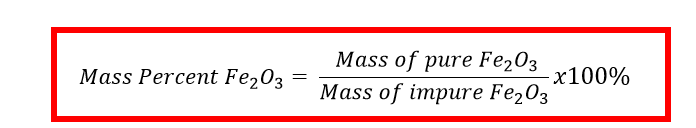# Problem: An iron ore sample contains Fe2O3 plus other impurities. A 752-g sample of impure iron ore is heated with excess carbon, producing 453 g of pure iron by the following reaction:Fe2O3 (s) + 3 C (s) → 2 Fe (s) + 3 CO (g)What is the mass percent of Fe 2O3 in the impure iron ore sample? Assume that Fe 2O3 is the only source of iron and that the reaction is 100% efficient.

🤓 Based on our data, we think this question is relevant for Professor Cruz's class at USF.

###### FREE Expert Solution

The mass percentage of pure Fe2O3 can be computed using the equation:Since the reaction is 100% efficient this means that all Fe2O3 in the iron ore reacted to form pure Fe.

The mass of impure Fe2Oor the mass of the iron ore sample is already given as 752 g

But, the mass of pure Fe2Oneeds to be calculated based on the moles of Fe produced in the reaction.

We can solve for the Mass of Fe2O3 through the following steps:

1. mass to mole calculation (converting mass of Fe to moles)
2. mole to mole calculation (moles Fe to moles Fe2O3 )
3. mole to mass calculation (converting moles to mass of Fe2O3)###### Problem Details

An iron ore sample contains Fe2O3 plus other impurities. A 752-g sample of impure iron ore is heated with excess carbon, producing 453 g of pure iron by the following reaction:

Fe2O3 (s) + 3 C (s) → 2 Fe (s) + 3 CO (g)

What is the mass percent of Fe 2O3 in the impure iron ore sample? Assume that Fe 2O3 is the only source of iron and that the reaction is 100% efficient.